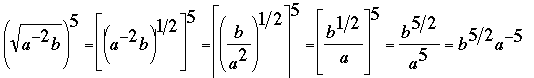SEARCH HOMEMath Central Quandaries & QueriesHow do I write the following in exponential form? ( square root of a^-2 b)^5 Thanks MandyHi Mandy,Since the square root can be written in exponential form as a 1/2 power you can write this asPennyMath Central is supported by the University of Regina and The Pacific Institute for the Mathematical Sciences.